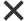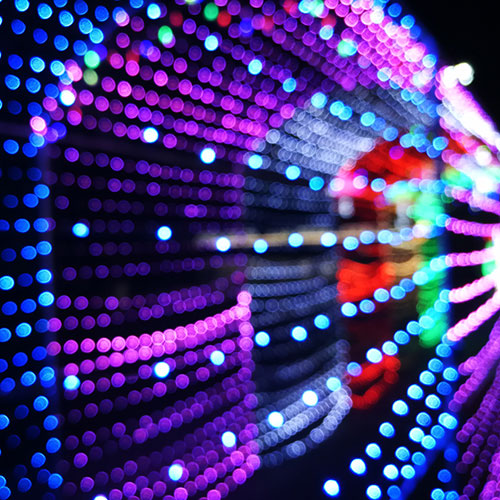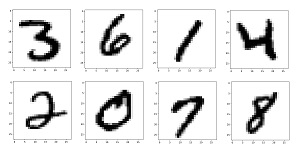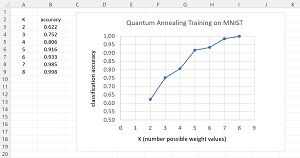News

### Researchers Explore Neural Network Slot Machine Technique Training

"Slot machine and quantum annealing training techniques might be useful in scenarios where neural networks must be retrained frequently and in scenarios where training must occur on devices with limited processing power, such as mobile phones," says Microsoft AI scientist engineer.Training deep neural networks is difficult, time-consuming and expensive. Researchers are actively exploring techniques to improve neural network training. One interesting effort is described in the paper "Slot Machines: Discovering Winning Combinations of Random Weights in Neural Networks" by M. Aladago and L. Torresani.

The idea of the slot machine technique training for neural networks is best explained by a concrete example. The MNIST (Modified National Institute of Standards and Technology) dataset consists of crude 28 x 28 (784 pixels) images of handwritten "0" to "9" digits. Each pixel value is a number between 0 (white) and 255 (black), and intermediate values representing shades of gray. Figure 1 shows some typical MNIST images.[Click on image for larger view.] Figure 1: Eight Example MNIST Images

A simple neural network classifier to identify an unknown MNIST digit could have a 784-1000-10 architecture: 784 input nodes (one for each pixel), 1000 hidden processing nodes, and 10 output nodes (one for each possible digit). In practice, neural image classifiers are more complex.

For a given set of 784 input pixel values, the output of a 784-1000-10 network might be 10 values that look like [0.12, 0.03, 0.65, 0.01, 0.08, 0.02, 0.04, 0.01, 0.03, 0.01]. The output values loosely represent the probabilities of each possible digit: "0," "1," "2," . . "9" and so the predicted digit would be a "2" because it has the highest pseudo-probability.

A 784-1000-10 neural network has (784 * 1000) + (1000 * 10) = 784,000 + 10,000 = 794,000 numeric variables called weights, plus 1,010 special types of weights called biases. You can think of a neural network as a very complicated math function where the values of the weights and biases, along with the input values, determine the output of the neural network.

Computing the values of a neural network's weights and biases is called training the network. Training an MNIST classifier uses a set of data that has known input pixel values and known correct digit values. Almost all neural network training uses some form of what's called stochastic gradient descent.

Each neural network weight and bias has an associated internal variable called a gradient. A gradient is just a numeric value, often between about -6.0 and +6.0. The value of a gradient determines how much to adjust its corresponding weight so that the network's computed output values are closer to the true, correct output values in the training data (or equivalently, so that output error is reduced, hence the "descent" in the name).

Stochastic gradient descent training is an iterative process that adjusts the weights and bias values slowly. For large neural networks with millions of weights and biases, gradient based training can take hours or days, even on powerful computing hardware. Therefore, researchers have explored many alternatives to stochastic gradient descent techniques.

The Slot Machine Training Technique
The slot machine technique training uses a radically different approach than stochastic gradient descent. In slot machine technique training, each neural network weight and bias gets a list of K = 8 possible values. These values are mathematically random. The training process consists of finding one of the eight possible values for each weight and bias.

Suppose a dummy neural network has just three weights, w1, w2, w3, instead of many thousands. And suppose each weight has just K = 4 possible values instead of the standard 8: w1 is one of [-2.6, 1.3, 0.7, -1.5], w2 is one of [1.8, -2.1, 0.3, 0.9], w3 is one of [-1.4, 0.9, 1.1, -0.6]. The slot machine technique would search through the 4 * 4 * 4 = 64 possible permutations of weight values to find the one permutation that gives the best results (lowest error) on the training data. The result might be [w1, w2, w3] = [1.3, -2.1, 1.1].

The challenge in slot machine technique training is searching through the permutations of possible weight values. If a simple neural network for the MNIST handwritten digits image dataset has 800,000 weights, and each weight has K = 8 possible values, then there are 8 * 8 * 8 * . . . 8 (800,000 times) permutations, which is a number so huge it's quite literally beyond comprehension.

The weight search algorithm described in the slot machine training research paper is an iterative technique called straight-through gradient estimation. Each possible weight value gets an associated score that measures how good the weight value is. On each iteration, the weight with the highest score is analyzed, and all scores are updated. When the algorithm finishes, the weights with the highest scores are used to define the trained model.

This approach is called a slot machine technique because each weight value can be just one of a set of discrete values. This is similar to an old slot machine where each reel can be just one from a set of discrete values such as "cherry," "7," "lemon" and so on.

The Quantum Annealing Technique
Researchers at Microsoft have explored a neural network training technique that is closely related to the slot machine technique. The technique is called quantum annealing training. Like the slot machine technique, quantum annealing training sets up K random possible values for each weight. But instead of using straight-through gradient estimation for weight scores, quantum annealing training uses a variation of simulated annealing.[Click on image for larger view.] Figure 2: Classification Accuracy on the MNIST Dataset Using Quantum Annealing

Simulated annealing is a classical machine learning technique for combinatorial optimization -- finding the best possible permutation of a set of discrete values. Quantum annealing enhances standard simulated annealing by using one or more ideas from quantum mechanics, such as quantum tunneling and quantum spin. Quantum annealing uses standard hardware and software, not exotic quantum computing hardware and software.

Experiments on the MNIST data set using quantum annealing give similar results to those obtained by experiments using the slot machine training technique. The graph in Figure 2 shows that as K, the number of possible values for each weight, increases from 2 to 8, classification accuracy increases from just over 60 percent to 99.8 percent which is the about the same accuracy obtained by stochastic gradient descent techniques.

What's the Point?
The Pure AI editors spoke to Dr. James McCaffrey, a scientist engineer at Microsoft Research who worked on the neural network quantum annealing training experiments. McCaffrey commented, "Standard deep neural network training with stochastic gradient descent techniques is effective in most scenarios."

McCaffrey continued, "For example, an elevator company could train an image classification model on the thousands of parts field technicians might encounter in the field. Training could occur offline because new parts are only rarely added to the company inventory."

McCaffrey speculated, "But the slot machine and quantum annealing training techniques might be useful in scenarios where neural networks must be retrained frequently and in scenarios where training must occur on devices with limited processing power, such as mobile phones."